# The solution idea to answer the RH

## Transcription

The solution idea to answer the RH
```The solution idea to answer the RH
Klaus Braun
Oct. 15, 2014
www.riemann-hypothesis.de
The Riemann Hypothesis states that the non-trivial zeros of the Zeta function all have real part
one-half. The Hilbert-Polya conjecture states that the imaginary parts of the zeros of the Zeta
function corresponds to eigenvalues of an unbounded self adjoint operator.
In [BeM] some evidence is presented that the eigenvalues are energy levels, that is eigenvalues of
a hermitian quantum (“Riemann”) operator associated with the classical hamiltonian
Hcl ( x, p)  xp ,
where x is the (one-dimensional) position coordinate and y the conjugate momentum.
From [DeJ] 18, VII, we recall “The non-trivial zeros of Riemann's zeta function arise from inquiries
into the distribution of prime numbers. The eigenvalues of a random hermitian matrix arise from
inquiries into the behavior of systems of subatomic particles under the laws of quantum mechanics.
What on earth does the distribution of prime numbers have to do with the behavior of subatomic
particles?"
All attempts failed so far to represent the Riemann duality equation in the critical stripe as
convergent Mellin transforms of an underlying self-adjoint integral operator equation.
The solution idea to answer the RH is about this:
two self-adjoint, bounded (singular integral) operators with appropriate Hilbert space
domains to enable proof P1 and proof P2.
1
Proof 1
The Poisson summation formula applied to the Gauss-Weierstrass density function
f ( x) : ex
2
enables the proof of the Jacobi’s   relation xG( x)  G(1 / x) for the Theta function

G ( x) : 1   ( x 2 ) :  f (nx) .

Let M denote the Mellin transform then it holds
M  f ( s ) 
1 s / 2 s .

( )
2
2
The natural statement of the functional equation is the symmetric duality equation ([EdH], 1.6)
(*)
 (s)M  f (s)   (1  s)M  f (1  s) .
Multiplying by s( s  1) and putting
1
2
s
2
 ( s) : s( s  1) ( s) ( )  s / 2
leads to the Riemann duality equation in the form
 (s)   (s)(s 1)M xf ( x)(s)   (1  s) , s  C .
Due to the corresponding property of the Hilbert transform the functions f , f H are identical in a
weak L2 (, )  sense, i.e. it holds
f
2
0
 fH
2
0

1
2
respectively
( f ,  )  ( f H ,  )   L2 (, ) .
From this it follows the identity
Fs : M  f (s)  M  f H (s) : FsH
in the sense of distributional complex-valued functions ([PeB] I.15).
2
There is an only formally valid representation of Riemann’s duality equation as transform of an
integral operator in the form ([EdH], 10.3):
(**)  (s)  s  1 x1 sG( x) dx   x1 sG( x) dx  .

s  1 2 0

x

x
1
This operator has no transform at all, as the integral
1
x
0
1 s

dx
dx
1
1
 x1 s  formally , only (!) 

0
x 1
x
1 s 1 s
does not converge for any s. The integral (**) would converge if the constant term
f (0)  fˆ (0)  1
is absent.
In order to overcome this issue to build series representation of  (s) as an even function of
Riemann introduced the auxiliary function ([EdH] 10.3)
H ( x) :
s  1/ 2 ,
d  2 d

x
G ( x)
dx  dx

which fulfills

2 ( s)   x1 s H ( x)
0
dx .
x
The Hilbert transform “spirits away” the jeopardizing constant Fourier series term ([PeB] Example
9.11)], i.e. the related Theta function G H has a vanishing constant term.
The Hilbert transform of the function f (x) is given by ([GrI] 3.952, 7.612, 9.21-9.23)

f H ( x)  4  f ( ) sin(2x)d
0
1 3
f H ( x)  4xf ( x)1 F1 ( ; ,x 2 )
2 2
f H ( x) 
4
x
e
1
 x 2
2
M 1 1 (x 2 ) .
,
4 4
It holds


dx
s
s
M  f H ( s)   x f H ( x)
  (1 s ) / 2 tan( )( )   3 / 2
x
2
2
0
s
tan( s)
2 M xf ( x)( s)   3 / 2

2
s

1
M  f ( s)  c tan( (1  s))M  f H ( s)  M xf ( x)( s)
2
s
3
s 0
The key idea is to replace

s
 x f ( x)
0


dx
1 s
dx
1 3
   s / 2 ( )   x s f H ( x)
 4  x s f ( x)1 F1 ( ; ; x 2 )dx
x
s 2
x
2 2
0
0
and
G ( x)  G H ( x )
to build a modified functional equation, being valid in a weak sense, but with same set of Zeta
function zeros.
With density arguments in combination with Hardy ‘s theorem ([EdH] 11.1), (the number of zeros of
the Zeta function on the critical line is infinite) the weak functional equation is then also valid in a
strong sense.
The prize to be paid is a RH analysis in a weak L2 (, )  Hilbert space framework and (weak)
variational representations of affected functions and dual integral operator equations. As this is
anyway the natural framework to deal with singular integral operators the prize seems to be more
than adequate.
For

1 3
1 ( x) n
F ( x) :1 F1 ( ; , x)  
2 2
n!
0 2n  1
it holds
xF ( x) 
1  2n (  x ) n 1  x
 e

2 0 2n  1 n!
2
and therefore ([GrI] 7.612)
s
1
s
M F ( ) 
( )
2
1 s 2
for 0  Re( s)  1
s
s
s
M xF ( x)( ) 
( )
2
s 1 2
for 0  Re( s)  1 .
From [SeA] we note that all zeros z n of

1 3
1 z n 1 zt 1/ 2
, z)  
  e t dt  z 3 / 4 e x / 2 M 1 1 ( z )
1 F1 ( ;
,
2 2
2
n

1
n
!
20
n 0
4 4
1
lie in the half-plane Re( z )  1 / 2 and in the horizontal stripe (2n  1)  Im( z )  2n .
With respect to [PoG1] we note that
z

2
1 3
z
4zf ( z )1 F1 ( ; , z 2 )  czez  (1  )e n
2 2

1
n
is a function of the Laguerre-Polya class, i.e. a function of increased genus 1.
4
Proof 2
The same idea of proof 1 can also be applied to the fractional part function in an alternative Hilbert
space framework ([KBr]):
Let H  L*2 () with  : S 1 ( R 2 ) , i.e.  is the boundary of the unit sphere. Let u (s) being a
2  periodic function and  denotes the integral from 0 to 2 in the Cauchy-sense. Then for
u  H : L2 () with  : S 1 ( R2 ) and for real  Fourier coefficients and norms are defined by
u :
1
u ( x)eix dx
2 
u
2


:  
2
u

2
.
Then the Fourier coefficients of the convolution operator
( Au )( x) :  log 2 sin
x y
u ( y)dy :  k ( x  y )u ( y)dy
2
and D( A)  H A  H 1 / 2 () .
are given by
( Au )  k u 
The operator
A
1
u .
2
enables characterization of the Hilbert spaces H 1 / 2 and H 1 in the form

H 1 / 2   
2
1 / 2


 ( A , ) 0   , H 1   
2
1

 ( A , A ) 0  
.
From ([BeB] 8, Entry 17 (iv)) we quote
“Ramanujan informs us to note that

cot(x)  2 sin(2 x)
,
1
which also is devoid of meaning, .... may be formally established by differentiating the equality

2
1
cos 2 x

5
 2 log 2 sin(x)
.
Putting

 ( x) : 2 ( x  x)     sin 2nx
1
2
n

2
n
 H ( x) : 2 log 2 sin(x)   cos 2nx
1

 : cot( )  2 sin(2 )
1
it holds (see also [ZyA] XIII, (11-3))

( A )( x)  


1
sin(2x)
 ( x )  
 H 0#
2

1
i.e.
  H #1 / 2
with

1 / 2
 
0
 H
0
.
The prize to be paid is a RH analysis in a weak H #1 / 2 (0,1)  Hilbert space framework ([PeB] I, §15,
appendix) and (weak) variational representations of affected functions and dual integral operator
equations (which is anyway the natural framework to deal with singular integral operators and
spectral theory):
In a weak H #1 / 2 (0,1)  sense it holds
 ( s)( s) cos(

2
s)  M  log 2 sin( )( s)   ( s) M cos ( s)
for 0  Re( s)  1
The relationship to the Riemann duality equation is given by ([TiE] 2.1)
2
M cos ( s) :  ( ) 1 s  (1  s)

with
 (1  s)  (s)  1
and
 (s)   (s) 1  (s) .
6
Proof 3+ proposals based on alternative Li-function
The Li-function is defined by ([GrI] xxxviii)
li ( x)  Ei (ln x)
x 1
with

Ei ( x)    e t
x

dt
:   d
t
x
.
According to P1 we define alternatively

1 3
2 2
 H ( x) : F ( x)1F1 ( ; , x)  
n 0
1 ( x) n
2n  1 n!
.
As it holds
2n (  x ) n
 e x
n!
n  0 2n  1

 2 xF ( x)  
this motivates the following alternative definition


EiH ( x) : 2 dF  
x
1
1
1
n
2
( x) n
n!
.
We claim that the related Li-function overcomes current challenges to prove the RH by related “Lifunction convergence” criteria.
7
Proofs 4+ proposals based on P1/P2 Hilbert space frameworks
The degenerated hypergeometric function allows the definition of an alternative series
representation of the Zeta function, alternatively with respect to Riemann’s Zeta function and to
Polya’s Zeta fake function, ([EdH], 1.8, 12.5):

 ( z )  2 (u ) cos(( zu)du .
0
It enables the application of corresponding Polya criteria ([PoG]) for other proofs of the RH.
In the context of the odd (!) function
2
1 3
xe x 1 F1 ( ; , x 2 )
2 2
we refer to the approach in [CaD] (based on [PoG1]) in the framework of Laguerre-Polya class
functions with special functions of genus >1 and its Weierstrass factorization form. The results of
[SeA] provides the corresponding nonzero real numbers condition that

1
1


2
 .
The Hilbert transforms of P1 and P2 define appropriate kernels of integral operators with
corresponding domains and ranges defined in appropriate Hilbert spaces. The corresponding
orthogonal systems of the related Hilbert spaces enable the definition of alternative Fourier integral
representations of the Zeta function itself. For the integral operators of P1 and P2 the following
polynomial systems are proposed:
P1: The Hilbert transformed Hermite polynomials
P2: The Lommel polynomials ([DDi], [WaG] 9-7).
8
References
[AmT] Amdeberhan T., Coffey M. W., Espinosa O., Koutschan C., Manna D. V., Moll V. H,
Integrals of Powers of Log-Gamma, to appear in Proc. Amer. Math. Soc.
[BaB] B. Bagchi, On Nyman, Beurling and Baez-Duarte’s Hilbert space reformulation of the
Riemann Hypothesis, Indian Statistical Institute, Bangalore Centre, (2005)
[BeB] Berndt B. C., Andrews G. E., Ramanujan´s Notebooks Part I, Springer Verlag, New York,
Berlin, Heidelberg, Tokyo, 1985
[BeM] Berry M. V., Keating J. P., H  xp and the Riemann zeros, in Super symmetry and Trace
Formulae: Chaos and Disorder, Ed. I.V. Lerner, J.P. Keating, D.E. Khmelnitski, Kluver, New York
(1999) pp. 355–367
[KBr] Braun K., Interior Error Estimates of the Ritz Method for Pseudo-Differential Equations, Jap.
Journal of Applied Mathematics, 3, 1, 59-72, (1986)
[CaD] Cardon D. A., Convolution operators and zeros of entire functions, Proc. Amer. Math. Soc.,
130, 6 (2002) 1725-1734
[DDi] D. Dickinson, On Lommel and Bessel polynomials, Proc. Amer. Math. Soc. 5, 946-956 (1954)
[DeJ] Derbyshine J., "Prime Obsession", Joseph Henry Press, Washington D.C., 2003
[EdH] Edwards H. M., Riemann´s Zeta Function, Dover Publications, Inc., Mineola, New York,
1974
[GrI] Gradshteyn I. S., Ryzhik I. M., Table of Integrals Series and Products, Fourth Edition,
Academic Press, New York, San Francisco, London, 1965
[HaH] Hamburger H., Über einige Beziehungen, die mit der Funktionalgleichung der
Riemannschen Zeta-Funktion äquivalent sind, Math. Ann. 85 (1922) pp. 129-140
[PeB] Petersen B. E., Introduction to the Fourier Transform and the Pseudo-Differential Operators,
Pitman Advanced Publishing Program, Boston, London, Melbourne, 1983
[PoG] Polya G., Über die Nullstellen gewisser ganzer Funktionen, Math. Zeit. 2 (1918), 352-383,
also, Collected Papers, Vol II, 166-197
[PoG1] Polya G., Über die algebraisch-funktionentheoretischen Untersuchungen von J. L. W. V.
Jensen, Mathematisk-fysiske Meddelelsev VII 17 (1927), 1-33
[SeA] Sedletskii A. M., Asymptotics of the Zeros of Degenerate Hypergeometric Functions,
Mathematical Notes, Vol. 82, No. 2 (2007) 229-237
[TiE] Titchmarsh E. C., The Theory of the Riemann Zeta-function, Clarendon Press, Oxford, 1951
[WaG] Watson G. N., A Treatise on the Theory of Bessel Functions, Cambridge University Press,
Cambridge, Second Edition first published 1944, reprinted 1996, 2003, 2004, 2006
[ZyA] Zygmund A., Trigonometric series, Vol. I, Cambridge University Press, 1968
9
Appendix
A.

 1 y
 y e sin(2xy )dy  
2
1 
2
xe x (
2
0

y
 1 y 2
e
cos(2xy )dy 
0
M sin ( s)  ( s) sin(
 1
2
)1 F1 (1 
 3
; ; x 2 )
2 2

, Re(  )  1 , [GrI], 3.952, 7.
, Re(  )  0 , [GrI], 3.952, 8.
1 2 
 1
 ( )1 F1 ( ; ;x 2 )
2
2
2 2

s) , M cos ( s)  ( s) cos( s)
2
2

in the critical stripe ([GrI] 3.952).
,
(1 - s) 
1
   ....
1- s
,
 ( s) 
1
1
  ....
s -1 2
.
 ( x)  x  x  x 
B. The functions
1  sin 2x
1


 ( x)
2 1 2 
2

 H ( x)   log 2 sin(x)  
1
cos 2x

are identical in a weak L#2 (0,1)  sense. It holds
i)
ii)
iii)

 ( s)
s

  x  s  ( x)
0
 ( s)( s) cos(

2
0
 H
2
0

2

for 0  Re( s)  1 , [TiE] 2.1
dx
x

s )   x s  H ( x)
0
2
dx
x
for 0  Re( s)  1
([AmT], [GrI] (4.224).
3
C. [EdH] 12.5, [PoG]: Let f ( x)  
a
1/ a
f (ux) F (u )du define a real self-adjoint operator (where
F (u ) is real and satisfy uF (u)  F (1/ u) ) which has the property that
u F (u ) is
nondecreasing on the interval 1, a  , then its Mellin transform has all its zeros on the critical
line.
10
D. In a H 1 / 2  sense ([PeB] I, §15) it holds for 0  Re( s)  1

x
s
 H ( x)
0

1  1
dx  1
   x s cos(nx)
 s
x
x
1 n 0
1 n

1
n
1
y s cos( y )
0

dy
dy

  ( s)  y s cos y
  ( s)( s) cos( s)
y
y
2
0
.
The Riemann duality equation is given by


.
2 ( s) :  ( s) s(1  s)   x s ( xf ( x))d log x   2 (1  s)
0

The idea for the proofs P1 and P2 are to replace
P1 :
P2 :
f ( x)  f H ( x )
,
s
1 
s
M  f ( s)   2 ( )  M  f H ( s)  
s
2
1 s
2
s

( ) tan( s)
2
2
 ( x)   H ( x) , M  (1  s)  2  (s  1)  M  H (1  s)   (s) in the critical stripe.
s 1
Note:
1.
M h(s)  (1  s)M h(s  1) , M xh(s)  sM h(s) , M ( xh)'(s)  (1  s)M h(s)
2. Hardy ‘s theorem, that the number of zeros of the Zeta function on the critical line is infinite,
provides the “transfer” density argument from weak to strong solution propositions.
3.
4.
M sin ( s)  ( s) sin(
f
2
0
 fH
2
0


2
1
2

s) , M cos ( s)  ( s) cos( s)
2
,

2
0
 H
2
0

in the critical stripe.
2
3
5 . [SeA]: All zeros z n of the Kummer function lie in the half-plane Re( z )  1 / 2 and in the horizontal
stripe
(2n  1)  Im( z )  2n .
6.
(1 - s) 
1
   ....
1- s
,
 ( s) 
1
1
  ....
s -1 2
.
E. For the linkage of variational theory to holomorphic function in the distribution sense we
recall from [BPe] chapter I §15 the
Definition: Let z  g z be a function defined on a open subset U  C with values in the
distribution space. Then g z is called a holomorphic in U  C (or g ( z ) : g z is called
holomorphic in U  C in the distribution sense), if for each   C c the function z  ( g s ,  ) is
holomorphic in U  C in the usual sense.
11
F. In the context of proof P2 we recall from [BaB]:
Proposition Let H denote the weighted l 2  space consisting of all sequences a  an n  N  of
complex numbers such that


n 1
n
an
2
 : 11,1,1,......
Let
with c1  n  c2 .
2
2

n
n
 n

 k :   ( ) n  1,2,3,....  H
 k

,
for k  1,2,3,...
and k be the closed linear span of  k . Then the Nyman criterion states that the following
statements are equivalent:
i) The Riemann Hypothesis is true
ii)   k .
G. [EdH] 1.8: The constant not vanishing Fourier term of



1
G( y ) :  f (ny )  1  2 f (ny ) : 1   ( y 2 )  y 1G( y 1 )
requires the definition of an auxiliary function ([EdH] 12.5)



H ( y ) : 2 yG ( y )  y 2 G ( y )  4  (ny ) 2 2 (ny) 2  3 f (ny )  y 1 H ( y 1 ) .
1
This leads to the representation

 ( s)   2(u ) cos(su)du
0
with
2(log x) :
x H ( x) ,
respectively

1
2
 ( s)   a 2 n ( s  ) 2 n
0
with

a 2 n : 4 x 3 / 4
1
1
( log x) 2 n
d x 3 / 2 ( x) dx .
2
(2n)!
dx
x

12

H. Putting

1 3
1 (  x) n
F ( x) :1 F 1( , ; x)  
2 2
n!
1 2n  1
It holds
 2 xF ( x)  e  x
and therefore for 0  Re( s)  1 / 2

( s )   x s e  x
0

(1  s)
dx
s
  * ( s) :  2 x s dF 2
 ( s )
1
x
1

2
s
0
s
2
respectively
( s )
 * ( s) .
s
 *
( s  1) (1  s)  (1  s)
13
```

### Calculus I Final, Sample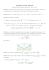More information

### A proof of the Riemann conjecture - Fuchs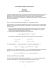More information

### Sample Solutions to Quiz 3 for MATH3270A − yMore information

### AS Entrance Examination Sample Paper Mathematics Instructions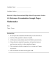More information

### Math 220 Sample Exam 4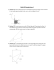More information

### Math 120 Sample questions for Chapters 4&5, with answersMore information

### Math 120 Sample questions for Test 2, with answers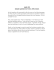More information

### 1.6 Exam advice and sample questions for Chapter 1More information

### Calculus II Final Sample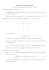More information

### Math 121 Sample Problems for Test 3 e 1. lim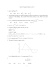More information

### Sample Question Paper for Class 12 CBSE 2013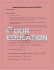More information